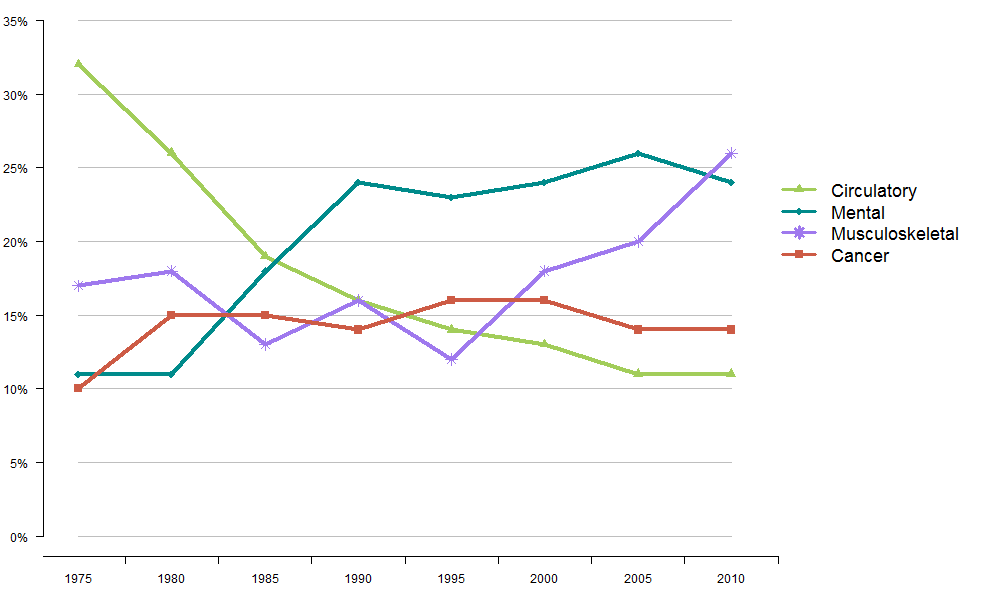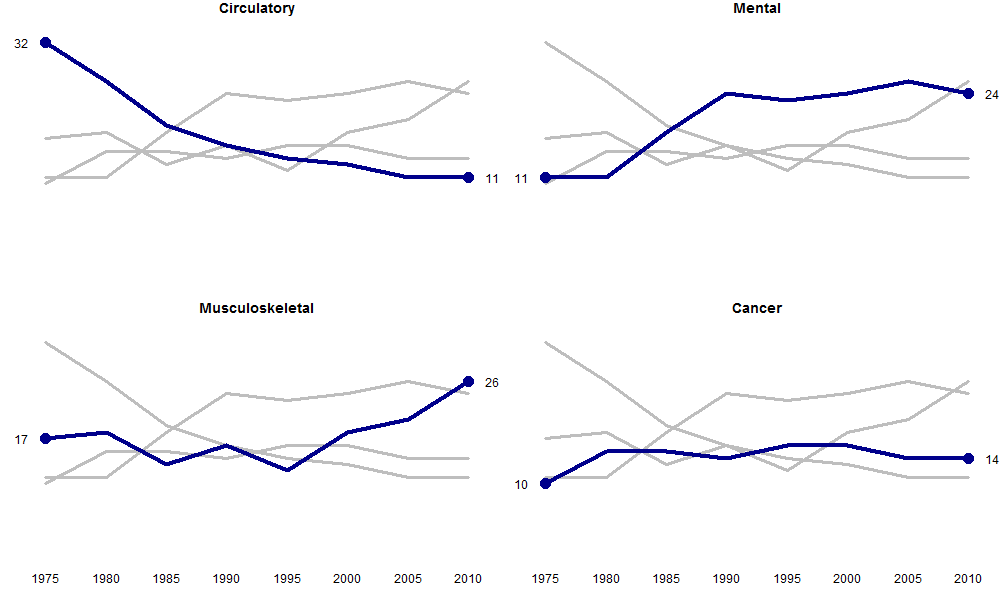Want to share your content on R-bloggers? click here if you have a blog, or here if you don't.

I have to admit to being a bit of a snob when it comes to graphs and charts in scientific papers and presentations.  It’s not like I think I am particularly good at it – I’m OK – it’s just that I know what’s bad.  I’ve seen folk screenshot multiple Excel graphs so they can paste them into a powerpoint table to create multi-panel plots… and it kind of makes me want to scream.   I’m sorry, I really am, but when I see Excel plots in papers I judge the authors, and I don’t mean in a good way.  I can’t help it.  Plotting good graphs is an art, and sticking with the metaphor, Excel is paint-by-numbers and R is a blank canvas, waiting for something beautiful to be created; Excel is limiting, whereas R sets you free.

Readers of this blog will know that I like to take plots that I find which are fabulous and recreate them.  Well let’s do that againI saw this Tweet by Trevor Branch on Twitter and found it intriguing:

It shows two plots of the same data.  The Excel plot:And the multi plot:You’re clearly supposed to think the latter is better, and I do; however perhaps disappointingly, the top graph would be easy to plot in Excel but I’m guessing most people would find it impossible to create the bottom one (in Excel or otherwise).

Well, I’m going to show you how to create both, in R. All code now in Github!

The Excel Graph

Now, I’ve shown you how to create Excel-like graphs in R before, and we’ll use some of the same tricks again.

First we set up the data:

```
# set up the data
df <- data.frame(Circulatory=c(32,26,19,16,14,13,11,11),
Mental=c(11,11,18,24,23,24,26,23),
Musculoskeletal=c(17,18,13,16,12,18,20,26),
Cancer=c(10,15,15,14,16,16,14,14))

rownames(df) <- seq(1975,2010,by=5)

df

```

Now let's plot the graph

```
# set up colours and points
cols <- c("darkolivegreen3","darkcyan","mediumpurple2","coral3")
pch <- c(17,18,8,15)

# we have one point on X axis for each row of df (nrow(df))
# we then add 2.5 to make room for the legend
xmax <- nrow(df) + 2.5

# make the borders smaller
par(mar=c(3,3,0,0))

# plot an empty graph
plot(1:nrow(df), 1:nrow(df), pch="",
xlab=NA, ylab=NA, xaxt="n", yaxt="n",
ylim=c(0,35), bty="n", xlim=c(1,xmax))

for (i in seq(0,35,by=5)) {
lines(1:nrow(df), rep(i,nrow(df)), col="grey")
}

# for each dataset
for (i in 1:ncol(df)) {

points(1:nrow(df), df[,i], pch=pch[i],
col=cols[i], cex=1.5)

lines(1:nrow(df), df[,i], col=cols[i],
lwd=4)

}

axis(side=1, at=1:nrow(df), tick=FALSE,
labels=rownames(df))

axis(side=1, at=seq(-0.5,8.5,by=1),
tick=TRUE, labels=NA)

axis(side=2, at=seq(0,35,by=5), tick=TRUE,
las=TRUE, labels=paste(seq(0,35,by=5),"%",sep=""))

legend(8.5,25,legend=colnames(df), pch=pch,
col=cols, cex=1.5, bty="n",  lwd=3, lty=1)

```

And here is the result:The multi-plot

Plotting multi-panel figures in R is sooooooo easy!  Here we go for the alternate multi-plot.  We use the same data.

```
# split into 2 rows and 2 cols
split.screen(c(2,2))

# keep track of which screen we are
# plotting to
scr <- 1

# iterate over columns
for (i in 1:ncol(df)) {

# select screen
screen(scr)

# reduce margins
par(mar=c(3,2,1,1))

# empty plot
plot(1:nrow(df), 1:nrow(df), pch="", xlab=NA,
ylab=NA, xaxt="n", yaxt="n", ylim=c(0,35),
bty="n")

# plot all data in grey
for (j in 1:ncol(df)) {
lines(1:nrow(df), df[,j],
col="grey", lwd=3)

}

# plot selected in blue
lines(1:nrow(df), df[,i], col="blue4", lwd=4)

points(c(1,nrow(df)), c(df[1,i], df[nrow(df),i]),
pch=16, cex=2, col="blue4")

mtext(df[1,i], side=2, at=df[1,i], las=2)
mtext(df[nrow(df),i], side=4, at=df[nrow(df),i],
las=2)

title(colnames(df)[i])

# add axes if we are one of
# the bottom two plots
if (scr >= 3) {
axis(side=1, at=1:nrow(df), tick=FALSE,
labels=rownames(df))
}

# next screen
scr <- scr + 1
}

# close multi-panel image
close.screen(all=TRUE)

```

And here is the result:And there we have it.

So which do you prefer?